﻿ 基于模拟–优化模式的梯级水库群突发水污染事件应急调度研究 Emergency Dispatching for Cascade Reservoirs of Sudden Water Pollution Accidents Based on the Model of Simulation and Optimization

Journal of Water Resources Research
Vol.07 No.02(2018), Article ID:24023,9 pages
10.12677/JWRR.2018.72018

Emergency Dispatching for Cascade Reservoirs of Sudden Water Pollution Accidents Based on the Model of Simulation and Optimization

Chengwei Yang1, Dingfang Li1, Dedi Liu2

1School of Mathematics and Statistics, Wuhan University, Wuhan Hubei

2School of Resources and Hydropower Engineering, Wuhan University, Wuhan Hubei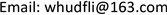Received: Feb. 20th, 2018; accepted: Mar. 6th, 2018; published: Mar. 13th, 2018ABSTRACT

According to characteristics and influences of sudden water pollution accidents, an emergency optimal operation model of simulation and optimization was established for cascade reservoirs based on hydrodynamic model and water quality model. The middle and lower reaches of Qingjiang River are selected as the case study. Applying a multi-objective optimization on the decision variables of the model (the control variables of emergency operating rules of QingJiang cascade reservoirs) by using improved NSGA-II algorithm results in the Pareto solutions and Pareto fronts and then the feasibility and effectiveness of emergency dispatching are discussed. The analysis results show that, the reservoirs close to the pollution source or directly connected with the polluted river should be called first in the capacity of reservoir storage. The time of reaching standard can be reduced by up to 80.4% when taking emergency dispatching. According to the optimization results, the competition between the energy lost in the emergency dispatch process and the time reaching standard is analyzed. The distribution of pollutant concentrations and the process of pollutant concentration changes with time are quantitatively simulated.

Keywords:Sudden Water Pollution Accidents, Cascade Reservoirs, Emergency Dispatching, Improved NSGA-II Algorithm, Pareto Solutions

1武汉大学数学与统计学院，湖北 武汉

2武汉大学水利水电学院，湖北 武汉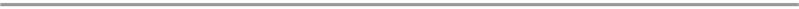1. 引言

2. 模型建立

2.1. 水动力模型

2.1.1. 马斯京根流量演算方程

${Q}_{ij}={C}_{0}^{i}\cdot {I}_{j}^{i}+{C}_{1}^{i}\cdot {I}_{j-1}^{i}+{C}_{2}^{i}\cdot {Q}_{j-1}^{i}$ (1)

2.1.2. 连续方程

$\frac{\partial A}{\partial t}+\frac{\partial Q}{\partial x}=q$ (2)

2.2. 水质模型

$\frac{\partial \left(CA\right)}{\partial t}+\frac{\partial \left(CQ\right)}{\partial x}=E\cdot \frac{\partial }{\partial x}\left(A\cdot \frac{\partial C}{\partial x}\right)-{K}_{1}\cdot C$ (3)

2.3. 应急调度优化模型

2.3.1. 目标函数

$\mathrm{min}\text{\hspace{0.17em}}\text{\hspace{0.17em}}{t}_{\text{end}}$ (4)

$\mathrm{min}\text{\hspace{0.17em}}\text{\hspace{0.17em}}{E}_{\text{waste}}=\underset{s=1}{\overset{n}{\sum }}{E}_{\text{extral-ideal}}^{\left(s\right)}-\underset{s=1}{\overset{n}{\sum }}{E}_{\text{extral-actual}}^{\left(s\right)}$ (5)

2.3.2. 调度函数

2.3.3. 约束条件

1) 污染物浓度达标约束：

$C\left(x,t\right)\le {C}_{s}\left(x\in {x}_{p}\right)$ (6)

2) s水库的水量平衡约束：

${V}_{s}\left(t\right)={V}_{s}\left(t-1\right)+\left[{\stackrel{¯}{I}}_{s}\left(t\right)-{\stackrel{¯}{Q}}_{s}\left(t\right)\right]\cdot \Delta t$ (7)

3) s水库的库容曲线约束：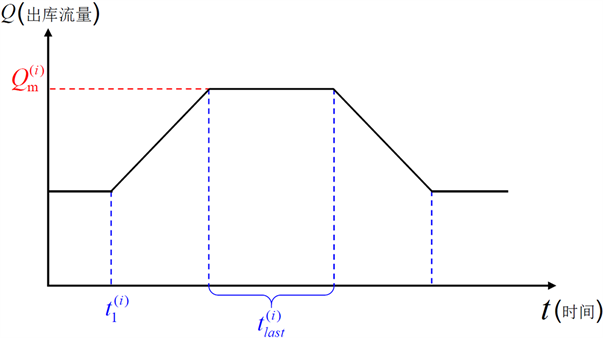Figure 1. Graph of reservoir operation

${Z}_{s,sy}\left(t\right)={f}_{ZV}\left[{V}_{s}\left(t\right)\right]$ (8)

4) s水库的库水位约束：

${Z}_{s,sy}^{\mathrm{min}}\le {Z}_{s,sy}\left(t\right)\le {Z}_{s,sy}^{\mathrm{max}}$ (9)

5) s水库的下游水位流量关系约束：

${Z}_{s,xy}\left(t\right)={f}_{ZQ}\left[{Q}_{s,ck}\left(t\right)\right]$ (10)

6) 水电站水头约束：

$\left\{\begin{array}{l}{H}_{s}^{\mathrm{min}}\le {\stackrel{¯}{H}}_{s}\left(t\right)\le {H}_{s}^{\mathrm{max}}\\ {\stackrel{¯}{H}}_{s}\left(t\right)=\frac{{Z}_{s,sy}\left(t-1\right)+{Z}_{s,sy}\left(t\right)}{2}-\frac{{Z}_{s,xy}\left(t-1\right)+{Z}_{s,xy}\left(t\right)}{2}-\Delta {H}_{s}\left(t\right)\\ \Delta {H}_{s}\left(t\right)={f}_{\Delta H,s}\left[{Q}_{s,fd}\left(t\right)\right]\end{array}$ (11)

7) 水电站出力约束：

${P}_{s}^{\mathrm{min}}\le {P}_{s}\left(t\right)\le {P}_{s}^{\mathrm{max}}$ (12)

2.4. 模型求解及程序实现

3. 实例研究

3.1. 水动力模型的率定与验证

3.2. 结果分析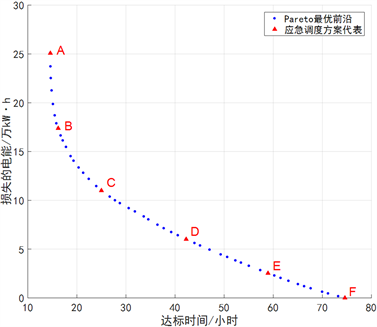Figure 2. Pareto front after optimizationFigure 3. Distribution of pollutant concentrations six hour after pollution incidentFigure 4. Concentration of phenol at confluence between QingJiang River and Yangtze River

3.3. 应急调度的高效益区间Table 2. Benefits and sacrifice of emergency dispatching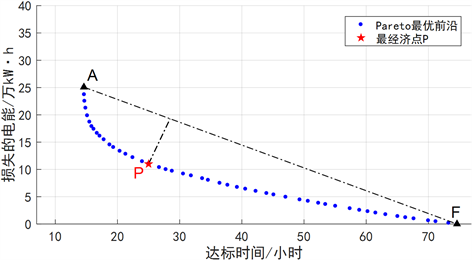Figure 5. Subset with high benefit of emergency dispatching scheme set

4. 结论

Emergency Dispatching for Cascade Reservoirs of Sudden Water Pollution Accidents Based on the Model of Simulation and Optimization[J]. 水资源研究, 2018, 07(02): 164-172. https://doi.org/10.12677/JWRR.2018.72018

1. 1. 郭生练, 陈炯宏, 刘攀, 等. 水库群联合优化调度研究进展与展望用[J]. 水科学进展, 2010, 21(4): 496-503. GUO Shenglian, CHEN Jionghong, LIU Pan, et al. State of the art review of joint operation for multi-reservoir systems. Advance in Water Science, 2010, 21(4): 496-503. (in Chinese)

2. 2. 雒文生, 李怀恩, 穆宏强. 水环境保护[M]. 北京: 中国水利水电出版社, 2008. LUO Wensheng, LI Huaien and MU Hongqiang. Water environmental protection. Beijing: China Water Power Press, 2008. (in Chinese)

3. 3. 王冰, 冯平. 梯级水库联合防洪应急调度模式及其风险评估[J]. 水利学报, 2011, 42(2): 218-225. WANG Bing, FENG Ping. Emergency operation model for cascade reservoirs and its risk evaluation. ShuiLi XueBao, 2011, 42(2): 218-225. (in Chinese)

4. 4. KACHIASHVILI, K., GORDEZIANI, D., LAZAROV, R., et al. Modeling and simulation of pollutants transport in rivers. Applied Mathematical Modelling, 2007, 31(7): 1371-1396. https://doi.org/10.1016/j.apm.2006.02.015

5. 5. PIMPUNCHAT, B., SWEATMAN, W. L., WAKE, G. C., et al. A mathematical model for pollution in a river and its remediation by aeration. Applied Mathematics Letters, 2009, 22(3): 304-308. https://doi.org/10.1016/j.aml.2008.03.026

6. 6. 王庆改, 赵晓宏, 吴文军, 等. 汉江中下游突发性水污染事故污染物运移扩散模型[J]. 水科学进展, 2008, 19(4): 500-504. WANG Qinggai, ZHAO Xiaohong, WU Wenjun, et al. Advection-diffusion models establishment of water pollution accident in middle and lower reaches of Hanjiang river. Advances in Water Science, 2008, 19(4): 500-504. (in Chinese)

7. 7. TANG, C. H., YI, Y. J., YANG, Z. F., et al. Water pollution risk simulation and prediction in the main canal of the South-to-North Water Transfer Project. Journal of Hydrology, 2014, 519: 2111-2120. https://doi.org/10.1016/j.jhydrol.2014.10.010

8. 8. TANG, C. H., YI, Y. J., YANG, Z. F., et al. Risk forecasting of pollution accidents based on an integrated Bayesian Network and water quality model for the South to North Water Transfer Project. Ecological Engineering, 2015, 96: 109-116.

9. 9. 练继建, 孙萧仲, 马超, 等. 水库突发水污染事件风险评价及应急调度方案研究[J]. 天津大学学报(自然科学与工程技术版), 2017, 50(10): 1005-1010. LIAN Jijian, SUN Xiaozhong, MA Chao, et al. Risk assessment and emergency dispatching of sudden water pollution incident in reservoir. Journal of Tianjin University (Science and Technology), 2017, 50(10): 1005-1010. (in Chinese)

10. 10. XIN, X.-K., YIN, W. and WANG, M. Reservoir operation schemes for water pollution accidents in Yangtze River. Water Science and Engineering, 2012, 5(1): 59-66.

11. 11. 王浩, 郑和震, 雷晓辉, 等. 南水北调中线干线水质安全应急调控与处置关键技术研究[J]. 四川大学学报(工程科学版), 2016, 48(2): 1-6. WANG Hao, ZHENG Hezhen, LEI Xiaohui, et al. Study on key technologies of emergency regulation and treatment to ensure water quality safety of the main canal of middle routes of South-to-North Water Diversion Project. Journal of Sichuan University (Engineering Science Edition), 2016, 48(2): 1-6. (in Chinese)

12. 12. 丁洪亮, 张洪刚. 汉江丹襄段水污染事故水库应急调度措施研究[J]. 人民长江, 2014(5): 75-78. DING Hongliang, ZHANG Honggang. Research on reservoir emergency regulation for treatment of sudden water pollution accident in Danjiangkou-Xiangyang reach of Hanjiang River. Yangtze River, 2014(5): 75-78. (in Chinese)

13. 13. 王家彪. 西江流域应急调度模型研究及应用[D]: [硕士学位论文]. 北京: 中国水利水电科学研究院, 2016. WANG Jiabiao. Research and application of reservoir system emergency operation model in Xijiang River Basin. Beijing: China Institute of Water Resources and Hydropower Research, 2016. (in Chinese)